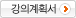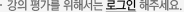### 주메뉴

### 확률과정론

• 경북대학교
• 홍순목
• 주제분류
공학 >전기ㆍ전자 >전기공학
• 강의학기
2016년 1학기
• 조회수
5,309
•
강의계획서This course provides a broad introduction to probability theory and random processes and their applications to engineering problems.

#### 차시별 강의11. Sample Space and Probability Set theory, Sample Space, Probability Axioms, Some Consequences of the Axioms.22. Discrete Random Variables Discrete Random Variables: PMF, CDF, Expectation, Variance and Standard Deviation, Joint PMF, Conditioning, Independence.33. Continuous Random Variables General Random Variables: CDF, PDF, and Gaussian RV's, Joint PDF, Continuous Conditioning.44. Further Topics on Random Variables Further Topics on Random Variables: Derived Random Variables, Covariance and Correlation, Conditional Expectation and Variance, Transforms, Sums of Independent Random Variables.55. Limit Theorems Limit Theorems: Markov and Chebyshev Inequalities, The Weak Law of Large Numbers, Convergence in Probability, The Central Limit Theorem.66. The Bernoulli and Poisson Processes Stochastic Processes: Bernoulli and Poisson Random Processes.77. Bayesian Statistical Inference Bayesian Statistical Inference: Point Estimation, Hyothesis Testing, MAP, Least Mean Square Estimation.88. Classical Statistical Inference Non-Bayesian Statistical Inference: Linear Regression, Binary Hypothesis Testing, Significance Testing.99. Classical Statistical Inference Non-Bayesian Statistical Inference: Linear Regression, Binary Hypothesis Testing, Significance Testing.#### 연관 자료#### 사용자 의견#### 이용방법

• 문서 자료 이용시 필요한 프로그램 [바로가기]

※ 강의별로 교수님의 사정에 따라 전체 차시 중 일부 차시만 공개되는 경우가 있으니 양해 부탁드립니다.